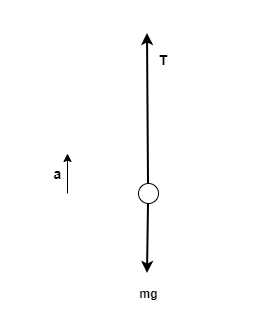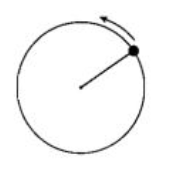# Problem: A ball on a string moves in a vertical circle, as shown. When the ball is at its lowest point, is the tension in the string greater than, less than, or equal to the ball's resting weight? Explain fully, using a free-body diagram and accompanying equations.

###### FREE Expert Solution

Newton's second law:

$\overline{){\mathbf{\Sigma }}{\mathbf{F}}{\mathbf{=}}{\mathbf{m}}{\mathbf{a}}}$

The free-body diagram at the lowest point is:81% (262 ratings)###### Problem Details

A ball on a string moves in a vertical circle, as shown. When the ball is at its lowest point, is the tension in the string greater than, less than, or equal to the ball's resting weight? Explain fully, using a free-body diagram and accompanying equations.